## The Eye Diagram¶

In this notebook, we are going to draw eye diagrams of different pulse shaping filters and evaluate, if they fulfill the first and second Nyquist criterion.

Let $g(t)$ be some pulse shaping filter which is used to pulse-shape transmit symbols in the baseband. When the (complex-valued) data symbols are given by $d[k]$, the overall transmit signal $x(t)$ is given by

$$x(t)=\sum_{k\in\mathbb{Z}}d[k]g(t-kT),$$

where $1/T$ is the symbol rate and $T$ is the time distance between adjacent symbols. We can understand the transmit signal as the superposition of time-shifted pulse shaping filters that are multiplied with the data-symbol. This technique is common to most communication systems.

Let us first define a function get_filter which returns different kinds of pulse shaping filters, depending on the parameters.

def get_filter(name, T, rolloff=None):
def rc(t, beta):
import warnings
with warnings.catch_warnings():
warnings.simplefilter("ignore")
return np.sinc(t)*np.cos(np.pi*beta*t)/(1-(2*beta*t)**2)
def rrc(t, beta):
return (np.sin(np.pi*t*(1-beta))+4*beta*t*np.cos(np.pi*t*(1+beta)))/(np.pi*t*(1-(4*beta*t)**2))

# rolloff is ignored for triang and rect
if name == 'rect':
return lambda t: (abs(t/T)<0.5).astype(int)
if name == 'triang':
return lambda t: (1-abs(t/T)) * (abs(t/T)<1).astype(float)
elif name == 'rc':
return lambda t: rc(t/T, rolloff)
elif name == 'rrc':
return lambda t: rrc(t/T, rolloff)


Let's draw the time-domain response for some of these filters to get a feeling about them:

T = 1
Fs = 100
t = np.arange(-3*T, 3*T, 1/Fs)
g = get_filter('rc', T, rolloff=0.5)  # RC filter with rolloff alpha=0.5
plt.figure(figsize=(8,3))
plt.plot(t, get_filter('rc', T, rolloff=0.5)(t), label=r'Raised cosine $\alpha=0.5$')
plt.plot(t, get_filter('rrc', T, rolloff=0.5)(t), label=r'Root raised cosine $\alpha=0.5$')
plt.plot(t, get_filter('rect', T)(t), label=r'Rectangular')
plt.plot(t, get_filter('triang', T)(t), label=r'Triangular', lw=2)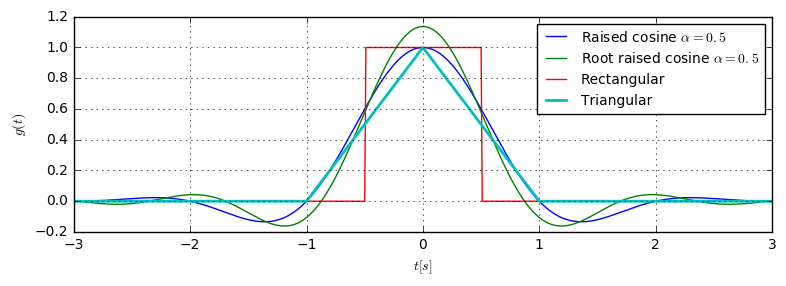We can now generate some random bits $b$ and modulate them with BPSK modulation, to get the data symbols $d$:

b = np.array([0, 1, 1, 0, 0, 1, 1, 0, 1, 0, 0, 1, 1, 0, 1, 0])
d = 2*b-1
print ("d=%s" % d)

d=[-1  1  1 -1 -1  1  1 -1  1 -1 -1  1  1 -1  1 -1]


With the modulated symbols, let us create the transmit signal according to the initially stated equation. Because we will use this operation several times, we wrap it into a reusable function get_signal.

def get_signal(g, d):
"""Generate the transmit signal as sum(d[k]*g(t-kT))"""
t = np.arange(-2*T, (len(d)+2)*T, 1/Fs)
g0 = g(np.array([1e-8]))
xt = sum(d[k]*g(t-k*T) for k in range(len(d)))
return t, xt/g0


Let's draw the signal for the given data sequence in thick black. Additionally, we can illustrate the several time-shifted pulse shapes (dashed blue):

fig = plt.figure(figsize=(8,3))
t, xt = get_signal(g, d)
plt.plot(t, xt, 'k-', lw=2, label='$x(t)$')
plt.stem(T*np.arange(len(d)), d)
for k in range(len(d)):
plt.plot(t, d[k]*g(t-k*T), 'b--', label='$d[k]g(t-kT)$')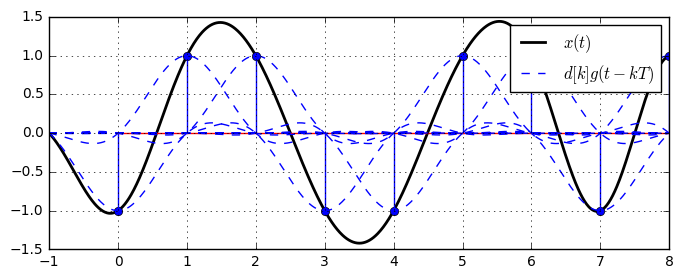From the transmit signal we can now draw the eye diagram. The eye diagram is obtained, when the transmit signal $x(t)$ is split into chunks of duration $2T$, where the start of all parts are $T$ apart. To illustrate this, let's draw the eye diagram step by step. The following function showEyeDiagramDrawing draws the transmit signal and highlights the part, which is currently used for drawing the eye diagram:

def showEyeDiagramDrawing(xt, T, partInd):
plt.subplot(211)
plt.plot(t, xt, 'k-', lw=1, label='$x(t)$') # Plot the overall signal

sigStart = 2*T*Fs  # ignore some transient effects at the beginning of the signal
samples_perT = Fs*T
samples_perWindow = 2*T*Fs
# extract the part of the signal we use for the current part of the eye diagram
sig_part = xt[sigStart + samples_perT*partInd + np.arange(samples_perWindow)]
t_emphasize = np.arange(2*T+T*partInd, 2*T+T*partInd+2*T, 1/Fs) + t.min()
# mark the part of the signal that currently contributes to the eye diagram
plt.plot(t_emphasize, sig_part, 'b-', lw=2)

plt.subplot(235)
t_part = np.arange(-T, T, 1/Fs)
# draw all parts of the eye diagram from previous signal portions in black
for p in range(partInd):
plt.plot(t_part, xt[sigStart + samples_perT*p + np.arange(samples_perWindow)], 'k-')
# draw the current part of the eye diagram in thick blue
plt.plot(t_part, sig_part, 'b-', lw=2)




As shown, the eye diagram is drawn step by step. We take the overall transmit signal and cut parts of length $2T$ out. Then, we draw all these parts into a single diagram, yielding the eye diagram of the filter.

The function drawFullEyeDiagram below uses the same technique to draw a full eye diagram without the time-consuming animation.

def drawFullEyeDiagram(xt):
"""Draw the eye diagram using all parts of the given signal xt"""
samples_perT = Fs*T
samples_perWindow = 2*Fs*T
parts = []
startInd = 2*samples_perT   # ignore some transient effects at beginning of signal

for k in range(int(len(xt)/samples_perT) - 6):
parts.append(xt[startInd + k*samples_perT + np.arange(samples_perWindow)])
parts = np.array(parts).T

t_part = np.arange(-T, T, 1/Fs)
plt.plot(t_part, parts, 'b-')

def drawSignals(g, data=None):
"""Draw the transmit signal, the used filter and the resulting eye-diagram
into one figure."""
N = 100;
if data is None:
data = 2*((np.random.randn(N)>0))-1
# fix the first 10 elements for  keeping the shown graphs constant
# between eye diagrams
data[0:10] = 2*np.array([0, 1, 1, 0, 0, 1, 0, 1, 1, 0])-1

t, xt = get_signal(g, data)

plt.subplot(223)
t_g = np.arange(-4*T, 4*T, 1/Fs)
plt.plot(t_g, g(t_g))

plt.subplot(211)
plt.plot(t, xt)
plt.stem(data)

plt.subplot(224)
drawFullEyeDiagram(xt); plt.ylim((-2,2))
plt.tight_layout()


Let us first draw the full eye diagram for the raised cosine filter with rolloff $\alpha=1$.

def showRCEyeDiagram(alpha):
g = get_filter('rc', T=1, rolloff=alpha)
drawSignals(g)Run code interactively  Hide
showRCEyeDiagram(alpha=1)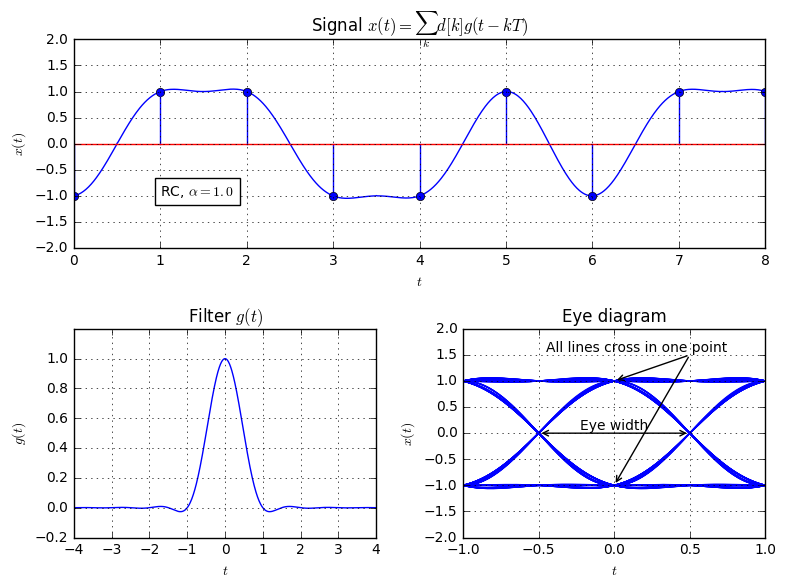In the eye diagram there are two annotations.

• If, at time $t=0$ all lines in the eye diagram that correspond to the same transmitted value $d[k]$ intersect at one point (namely $t=0$, $x(0)=d[k]$), the filter fulfills the First Nyquist Criterion, which describes that the filter is ISI-free. The first Nyquist Criterion is equivalently fulfilled, when the filter $g(t)$ has zero-crossings at the symbol times $t=nT, n\neq 0$. The RC filter with $\alpha=1$ is ISI-free.
• If zero-crossings of the lines only happen at time $t=0.5$, the filter fulfills the Second Nyquist Criterion. This corresponds to a maximum eye width of the diagram and allows maximum robustness against sampling offsets. The RC filter with $\alpha=1$ fulfills the second Nyquist Criterion.

Now, let's look at the eye diagrams of the RC filter with different rolloffs:




As we see, the RC filter always fulfills the First Nyquist Criterion. However, the 2nd Nyquist Criterion is only fulfilled for $\alpha=1$. In fact, the raised cosine filter with $\alpha=1$ is the only bandlimited filter that fulfills the 1st and 2nd Nyquist criterion.

In the following, let's look at the eye diagrams of several filters and evaluate, which Nyquist Criterions they fulfill. We start with the definition of some random data sequence $d$:

d = 2*np.array([0, 1, 0, 0, 1, 1, 0, 1, 0, 0, 0, 1, 1, 0, 1, 1, 0, 1, 0, 0, 0, 1, 0, 1, 1, 0, 1, 1, 1, 0, 1]) - 1
print ("d=%s" % d)

d=[-1  1 -1 -1  1  1 -1  1 -1 -1 -1  1  1 -1  1  1 -1  1 -1 -1 -1  1 -1  1  1
-1  1  1  1 -1  1]


First, let's evaluate the eye diagram of the root-raised cosine filter:

plt.figure(figsize=(8,6))
drawSignals(get_filter('rrc', T=1, rolloff=0.5), data=d)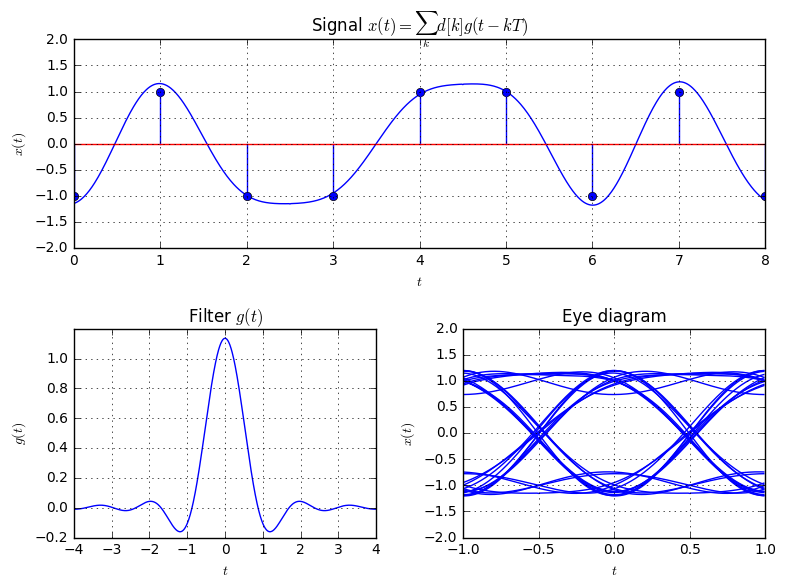Apparently, the RRC filter does neither fulfill the first nor the second: The lines do not cross in a single point at $t=0$, and also the zero-crossings are not exclusively at $t=0.5$.

Let's look at the eye diagram generated from a transmission of a simple triangular pulse:

plt.figure(figsize=(8,6))
drawSignals(get_filter('triang', T=1), data=d)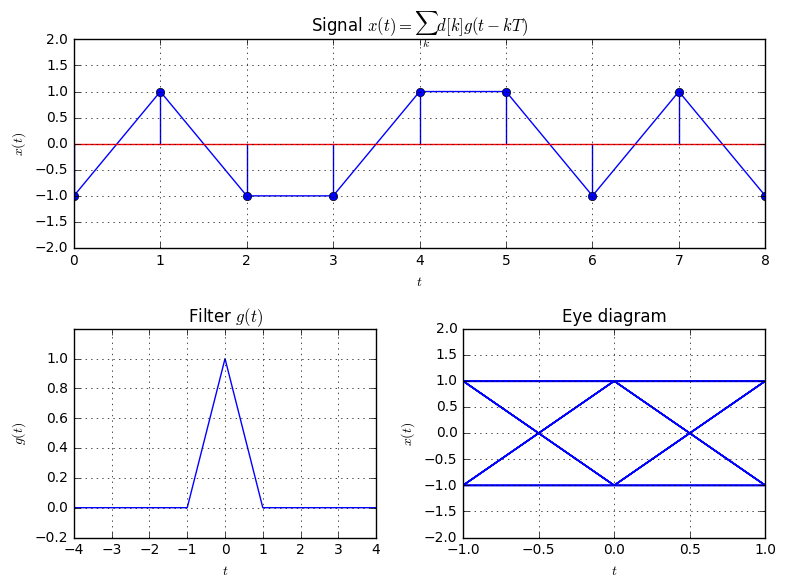As we see, both Nyquist-Criterions are fulfilled. Note that the triangular pulse has infinite bandwidth due to to the abrupt change in its slope at $t=\{-1,0,1\}$. Therefore, it is possible to fulfill both Nyquist-criteria (for bandlimited filters, the RC filter with $\alpha=1$ is the only filter that fulfills both criteria).

Now, let's have a look at a the eye diagram, when we use a triangle of half width compared to the previous figure:

plt.figure(figsize=(8,6))
drawSignals(get_filter('triang', T=0.5, rolloff=0.5), data=d)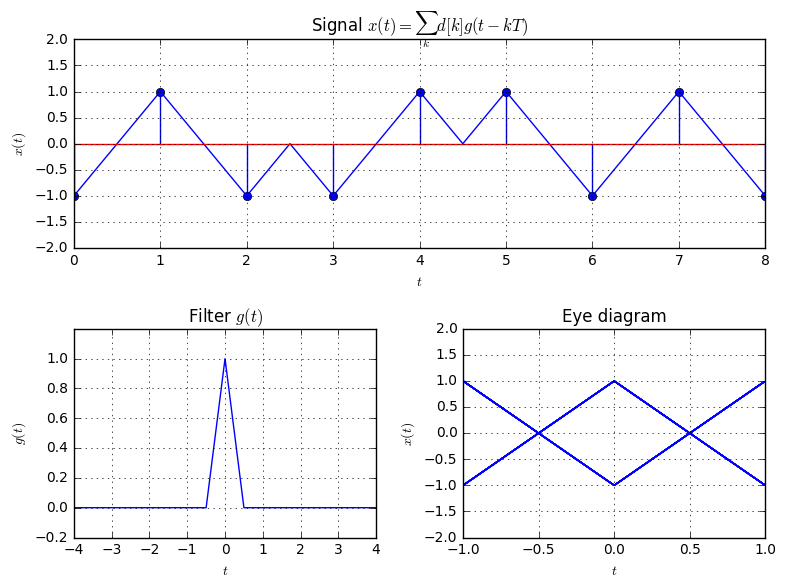Again, both Nyquist criteria are fulfilled. However, since the filter has half length compared to the previous one, it would occupy the double bandwidth.

Now, let's look at eye diagrams of rectangular filters, where the filter is either NRZ (non-return-to-zero, rect of width $T$) or RZ (return-to-zero, rect of width $T/2$):

plt.figure(figsize=(8,6))
drawSignals(get_filter('rect', T=1, rolloff=0.5), data=d)